# C++ program to sort strings

In this article, you will learn and get code to sort string(s) in C++. Here is the list of programs that sort string(s) entered by the user at run-time:

## Sort a string in ascending order in C++

This program sorts the string entered by the user in ascending order. For example, if the given string is bcabcz, then the same string in ascending order is abbccz. Let's have a look at the program:

The question is, "Write a program in C++ to sort a string in ascending order." Here is its answer:

```#include<iostream>
#include<stdio.h>
#include<string.h>
using namespace std;
int main()
{
char str, ch;
int i, j, len;
cout<<"Enter the String: ";
gets(str);
len = strlen(str);
for(i=0; i<len; i++)
{
for(j=0; j<(len-1); j++)
{
if(str[j]>str[j+1])
{
ch = str[j];
str[j] = str[j+1];
str[j+1] = ch;
}
}
}
cout<<"\nString in Ascending Order: "<<str;
cout<<endl;
return 0;
}```

This program was built and runs under the Code::Blocks IDE. Here is its sample run: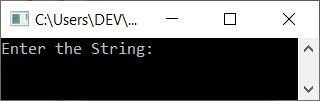Now supply the input, say "codescracker," as a string, then press the ENTER key to sort it in ascending order and print the sorted string on the output as shown in the snapshot given below: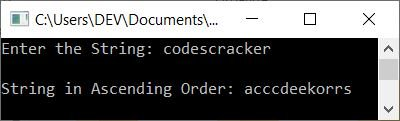The dry run of the above program with user input goes like this:

• When the user enters a string, then it gets stored in str in a way that:
• str=c
• str=o
• str=d
• and so on up until
• str=r
• Now, using the function strlen(), the length of the string gets initialized to the len variable. Because the length is 12, len=12.
• Now the execution of the for loop begins. That is, i=0 and the condition i<len or 0<12 evaluates to be true, therefore program flow inside the loop.
• Inside the loop, there is another for loop. So j=0 and the condition j<(len-1) or 0<11 evaluates to be true, therefore program flow goes inside the loop.
• Now using if, we compared the string at the current index using j with its adjacent index using j+1.
• That is, the condition (of if) str[j]>str[j+1] or str>str or c>o evaluates to be false, therefore program flow does not go inside the if's body. But whenever the condition evaluates to be true, program flow goes inside the body and swaps the character.
• Now the program flow goes to the update part of the inner for loop and increments the value of j. Now j=1, and the condition again gets evaluated.
• The condition j<(len-1) or 1<11 evaluates to be true again, therefore program flow goes inside the loop. This process continues until the condition evaluates to be false.
• When the inner for loop's evaluation is completed, the program flow moves to the outer for loop's update section and increments the value of i. So i=1, and the condition again gets evaluated.
• The condition i<len or 1<12 evaluates to be true again, therefore program flow goes inside the loop again. This process continues until the condition is evaluated as false.
• In this way, the string gets sorted in ascending order.
• So just print the value of str after exiting from the outer for loop.

Note: To manually find the length of a string without using the strlen() function, see the find length of a string article for all of the necessary code.

## In C++, sort the string in descending order

To sort the string in descending order, just change the following condition in the if statement:

`if(str[j]>str[j+1])`

with the code given below:

`if(str[j]<str[j+1])`

The entire program can be reversed with just a greater than (>) and less than (<) sign.

Here is its sample run with the same user input as the previous program, that is codescracker: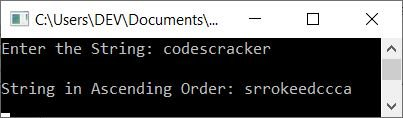## Sort the string (names) in alphabetical order

To sort strings (names) in alphabetical order in C++ programming, you have to ask the user to enter a few names (strings). Then sort these entered strings or names in alphabetical order as shown in the program given below:

This program only receives 5 names from the user at runtime. The question is: write a program in C++ to sort five names entered by the user in alphabetical order. Here is its answer:

```#include<iostream>
#include<string.h>
using namespace std;
int main()
{
char nameAll, nameOne;
int i, j;
cout<<"Enter 5 Strings (Names): ";
for(i=0; i<5; i++)
cin>>nameAll[i];
for(i=1; i<5; i++)
{
for(j=1; j<5; j++)
{
if(strcmp(nameAll[j-1], nameAll[j])>0)
{
strcpy(nameOne, nameAll[j-1]);
strcpy(nameAll[j-1], nameAll[j]);
strcpy(nameAll[j], nameOne);
}
}
}
cout<<"\nStrings (Names) in Alphabetical order:\n";
for(i=0; i<5; i++)
cout<<nameAll[i]<<endl;
cout<<endl;
return 0;
}```

Here is the initial output produced by this program: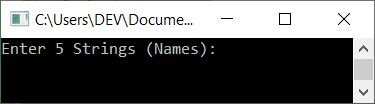Now, provide any five strings (names) and press the ENTER key to sort all five names alphabetically. Now print strings (arranged in alphabetical order) as shown in the output given below: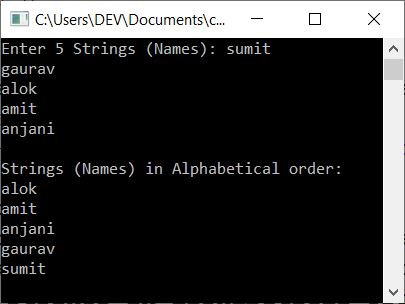#### Allow the user to customize the size of the names

Now this program allows the user to enter the size. That is how many names the user wants to enter. And then sort all those names in alphabetical order as shown here in the following program:

```#include<iostream>
#include<string.h>
using namespace std;
int main()
{
int i, j, tot;
char nameAll, nameOne;
cout<<"How many String to Enter (Max.50) ? ";
cin>>tot;
cout<<"Enter "<<tot<<" Strings (Names): ";
for(i=0; i<tot; i++)
cin>>nameAll[i];
for(i=1; i<tot; i++)
{
for(j=1; j<tot; j++)
{
if(strcmp(nameAll[j-1], nameAll[j])>0)
{
strcpy(nameOne, nameAll[j-1]);
strcpy(nameAll[j-1], nameAll[j]);
strcpy(nameAll[j], nameOne);
}
}
}
cout<<"\nStrings (Names) in Alphabetical order:\n";
for(i=0; i<tot; i++)
cout<<nameAll[i]<<endl;
cout<<endl;
return 0;
}```

Here is its sample run with user input of 7 names: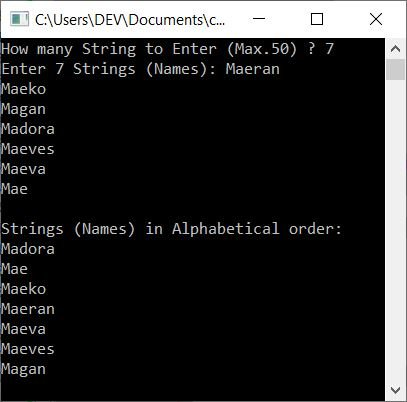#### The same program in different languages

C++ Quiz

« Previous Program Next Program »